Friday , August 23 2019

# What is Diode?

This post is about What is Diode? and Why we need Diode in Circuit. The diode controls direction of current flow in circuit. It is used for AC to DC conversion (rectification), in switching application. Simply, diode is one way gate in electronics to control current flow.

The simplest unidirectional passive semiconductor device is diode. Ideally, diode allows to flow current only in one direction, called as forward current. Diode has two distinct polarized terminals, they are anode and cathode. The forward current flows through diode from anode to cathode.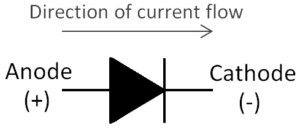Circuit symbol of Diode

### How Diode Works?

Diode operates in forward bias or in reverse bias mode. Let’s consider ideal diode first. When ideal diode is forward biased, it will short circuit (turn ON)and conducts current. When diode is reverse biased, it will open circuit (turn OFF) and no current flows through it. The VI characteristics of ideal diode is linear and is shown below.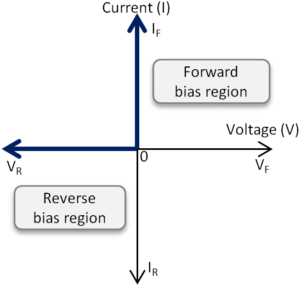VI Characteristic of Ideal Diode

There is not much difference in ideal and practical diode characteristics. Practically some power is consumed by diode during forward bias. It will not block all reverse current when reverse biased. This power consumed during forward bias is defined in terms of forward voltage. This voltage is also known as cut-in or knee voltage. It is ~0.7V for Silicon and ~0.3V for Germanium. The practical forward voltage varies with current, temperature and type of diode used. Let’s see practical diode characteristics with following examples.

#### Example 1 – Diode in forward bias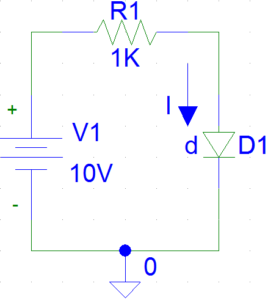Example of Forward Biased Diode

In forward bias, practical diode acts as a short circuit when input voltage is greater than forward voltage (VF ). During positive forward voltage, significant amount of current flows through diode called as forward current. The waveform below shows simulation of VI characteristics of forward biased diode. The current in forward bias is usually in miliamps. Here, resistor present between voltage source and diode minimizes current to microamps.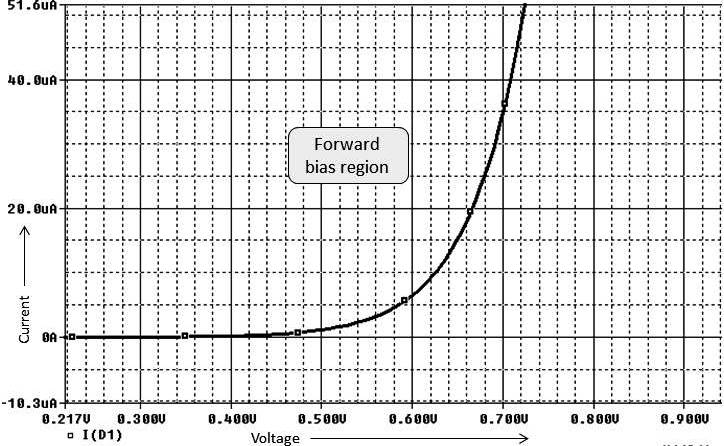VI Characteristics of Forward Biased Practical Diode

#### Example 2 – Diode in reverse bias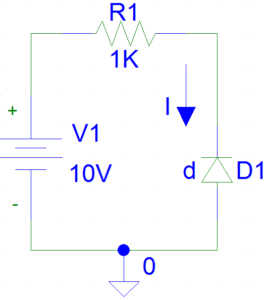Example of Reverse Biased Diode

In reverse bias, practical diode acts as an open circuit. Here voltage is less than VF but greater than large negative voltage called breakdown voltage (VBR ). The waveform below shows simulation of VI characteristics of reverse biased diode. During reverse bias, usually negligible amount of current flows through diode. If the reverse voltage exceed diodes breakdown voltage (VBR ), large amount of current flows through the diode destroying the diode junction.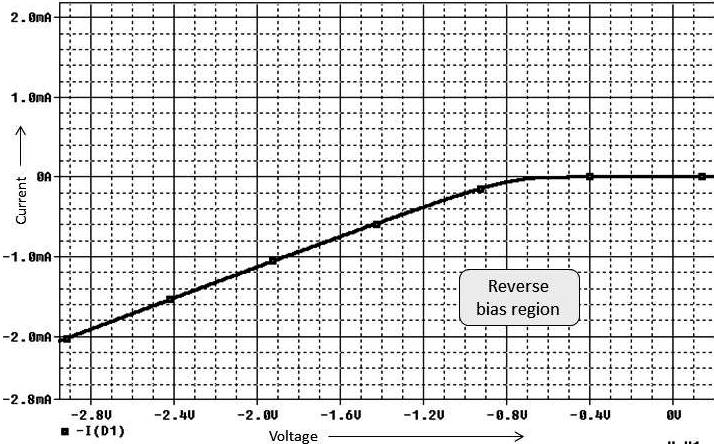VI Characteristics of Reverse Biased Practical Diode

### The diode datasheet

A datasheet of diode contains mechanical details, voltage and current ratings, working characteristic (VI curves) etc. These details have great significance in simulation, circuit and PCB design. Let’s take an example of 1N400X series diodes. You may google to get datasheet. This datasheet contains information of 1N4001 to 1N4007 diodes. The highlighted details have great significance while selecting diode.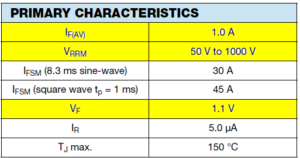Primary Characteristics of 1N400x Series Diode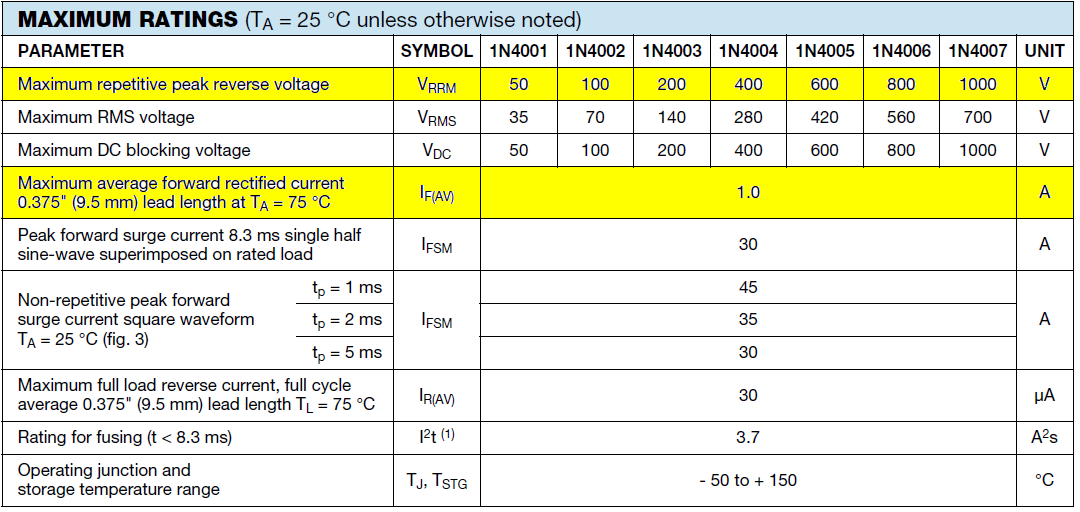Voltage and Current Ratings of 1N400X series Diode

I hope now you know what diode is and why we need diode in circuit. Diodes are key component of regulated power supplies, UPS and inverter circuits. In next post we’ll learn about different types of diode. Thanks for reading.

### About Roshankumar BhamareRoshankumar Bhamare received the Master degree in Electronics and Telecommunication engineering. He is currently a Lecturer in Engineering College. He is life member of Indian Society for Technical Education (ISTE). He is also a co-author of BINARYUPDATES.COM. His current interests include Electronics Design Automation (EDA) tools, Microcontroller based circuits.